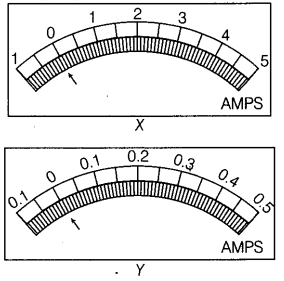# To measure the current, there is a choice of two ammeters X and Y

To measure the current, there is a choice of two ammeters X and Y. The scale on the face of each meter is shown in the following figures.Which meter would you choose, given that the temperature of the coil is always between 0°C and 100°C?

We would choose meter Y because the range of current to be measured is very small, i.e. 0 to 0.4 mA.
The meter Ywill show a largest possible deflection for a current within this range reducing the error in the measurement of the current.